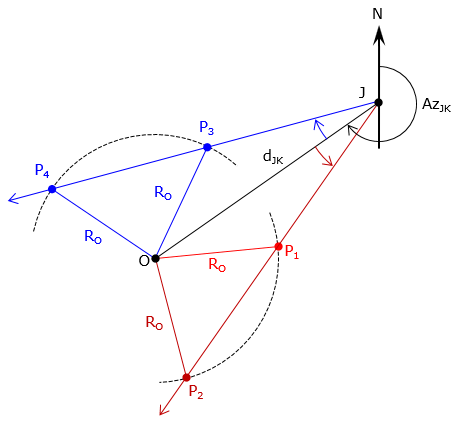### 1. Other types

Almost all other types of intersections are variations of distance-distance, direction-distance, and direction-direction.

#### a. Angle-distance

An angle-distance intersection, Figure F-1, is similar to direction-distance. The only difference is that angle-distance uses an angle and its turn direction from the base line. Otherwise, the solution process is the same.Figure F-1 Angle-distance intersection

The angle turn direction (right/left, clockwise/counterclockwise) must be provided to prevent an ambiguity. Figure F-2 shows four different intersection points (two to the left, two to the right) depending on the direction of the angle at point J.Figure F-2 Angle-angle ambiguity

#### b. Angle-angle

An angle-angle intersection, Figure F-3, is similar to a direction-direction intersection. Instead of directions to the intersection point, angles at both ends of the base line are included. Directions can be computed from these angles. The solution process is the same as a direction-direction intersection.Figure F-3 Angle-angle intersection

As with the angle-distance intersection, turn directions for each angle must be included. If turn directions are not included, there are four possible scenatoris, only two if which will result in an intersection, Figure F-4.(a) Angle J: (-) Angle K: (+)(b) Angle J: (+) Angle K: (-)(c) Angle J: (+) Angle K: (+) No intersection(d) Angle J: (-) Angle K: (-) No intersection Figure F-4 Angle turn directions

#### c. Arc-arc

An arc-arc (circle-circle) intersection, Figure F-5, is the same as is a distance-distance intersection. The radius point of each arc defines the base line and their respective radii are the two distances. As with a distance-distance intersection, there are two possible points and they would be solved for the same way.Figure F-5 Arc-arc intersection

#### d. Direction-arc

Figure F-6, is an direction-arc (direction-circle) intersection which is the same as a direction-distance intersection. A line at a specific direction starts at one end of the base line and intesects an arc centered on the other end.Figure F-6 Arc-line intersection

As in the angle-distance intersection, the angle turn direction must be defined otherwise we have the same situation as shown in Figure F-2. The solution process is the otherwise same as the distance-direction method.

#### e. Angle-arc

A line at a specific angle at one end of the base line intersects an arc centered on the other end of the base line. This is the same as the angle-distance intersection and like that type, the angle turn direction must be specified otherwise the ambiguous situation in Figure F-7 will result.Figure F-7 Angle-arc intersection